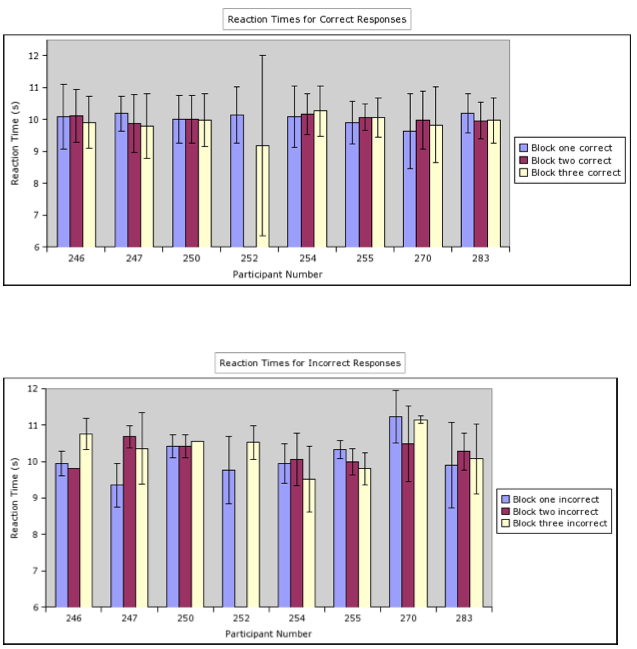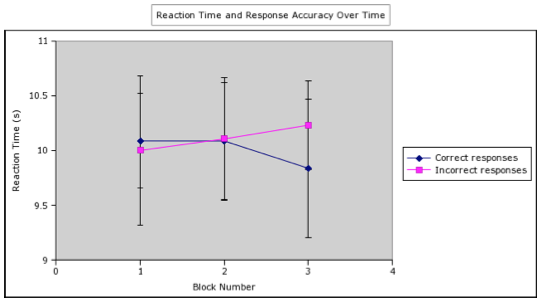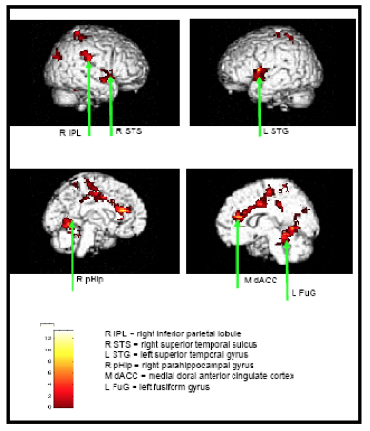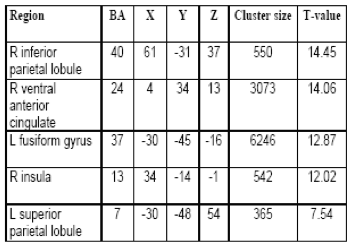# Individualized Behavioral and Image Analysis of Response Time, Accuracy, and Social Cognitive Load During Social Judgments in Adolescents

By
Discussions
2007, Vol. 2 No. 1 | pg. 3/4 |

### Response Time vs. Accuracy

Further analysis of the behavioral glove response data showed that participants made correct social judgments faster then they made incorrect social judgments. The mean reaction time for correct answers was 9.97s ± 0.23s while the mean reaction time for incorrect answers was10.23s ± 0.47s. These results are shown in figure 7.

Figure 7: This figure shows the average reaction times for correct and incorrect answers. The data indicates that correct answers had a faster reaction time then the incorrect answers. The data also indicates that incorrect answers had a wider variation of responses as compared to the correct responses.This finding demonstrates that the response time of the participant to the social stimuli was highly dependant on the participants' accuracy on the task. A t-test showed there to be a significant difference in reaction time for correct and incorrect answers, t(286), P = .000; t(87), P = .000. It is also of interest to note the differences in the variances between the correct and incorrect reaction times. While correct responses took roughly the same time across all blocks, incorrect response times had a greater variation. This is particularly clear in Figure 8. These figures are also important in that they illustrate that participants were neither getting more accurate or inaccurate as the study progressed.

Figure 8: a) This section of the figure shows the reaction times for correct answers for all participants across all blocks. b) This section of the figure shows the reaction times for incorrect reaction times for all participants across all blocks. These figures demonstrate that correct answers had a faster reaction time then incorrect answers. The data also indicates that there was greater variability of reaction times for incorrect answers then there was for correct answers.Another interesting item to note about the relationship between response time and accuracy is how it changes over time. Originally, correct and incorrect answers have about the same reaction time, with correct responses (m = 10.09s ± 0.43s) taking slightly longer then incorrect answers (m = 10.00s ± 0.68s). During the second block, the response times for correct and incorrect answers become nearly identical, with the response times of the incorrect answers (m = 10.11s ± 0.56s) increasing and the response times of the correct answers (m = 10.09s ± 0.53s) decreasing slightly. By the third block, the reaction times of the incorrect answers (m = 10.23s ± 0.41s) has continued to increase while the reaction times of the correct answers (m = 9.84s ± 0.63s) has decreased. The end result is that the response times of incorrect answers increase over time, while the reaction times of correct answers decrease over time. This data can be seen in figure 9.

Figure 9: This figure demonstrates the reaction times of correct and incorrect answers over time (across blocks). In the first block, correct answers took slightly longer to answer then incorrect answers. In the middle block, correct answers take about the same amount of time, with incorrect answers taking slightly longer to answer. By the third block, the incorrect answers take an appreciably longer amount of time to answers then correct answers. Overall, there is an inverse relationship between reaction time and accuracy and as time goes on it takes less time for participants to answer questions correctly.### Imaging Analysis

The imaging analysis previously completed by Ciccia and colleagues (2006) identified the following areas to have significant areas of activation during tasks of high social cognitive demand (represented by questions of type three) as compared to tasks of minimal social cognitive demand (represented by questions of type one) included: the left superior temporal gyrus (BA 22), the left anterior cingulated gyrus (BA 32), the left fusiform gyrus (BA 37), the right parahippocampal gyrus (BA 19), and the right inferior parietal lobule (BA 40). These results can be found in Table 1 and Figure 10.

Figure 10: This figure shows the areas of activation during tasks of high social cognitive demand as compared to tasks of moderate cognitive demand.Table 1: This table shows the areas of activation during tasks of high cognitive demand as compared to tasks of low cognitive.Significant areas of activation during tasks of high social cognitive demand (represented by questions of type three) as compared to tasks of moderate cognitive demands (represented by questions of type two) include the following a priori regions: the left fusiform gyrus (BA 37), the left inferior parietal lobule (BA 40), the right anterior cingulated gyrus (BA 24), and the right inferior parietal lobule (BA 40). These results can be seen in Table 2 and Figure 11.

Figure 11: This graph shows the areas of activation during a task of high cognitive demand as compared to tasks of low cognitive demand. These images were made using the traditional 10s time point as an average decision time.Table 2: This graph shows the areas of activation during a task of high cognitive demand as compared to tasks of low cognitive demand. These images were made using the traditional 10s time point as an average decision time.There were no significant areas of activation identified for the tasks of moderate social cognitive demand ( represented by the questions of type two) as compared to tasks of a minimal cognitive demand (represented by questions of type one).

The imaging analysis completed for the present study yielded different results from those found by Ciccia and colleagues (2006). In the analysis for the present study, the scans representing the actual decision times were compiled and input into the SPM software. The most accurate and most inaccurate participants data were analyzed. These analyses demonstrate that there is a difference in activation between these two methodologies. Figure 12 demonstrates these drastic differences between the two methodologies.

Figure 12: This figure shows the differences between the two methodologies. The images on the left were created using the exact reaction time methodology. The images on the right are those created by the original 10s method used by Ciccia and colleagues (2006). The images represent the same decisions, and should be the same if the two methods were equal. The top two images come from the most accurate participant and the bottom two images come from the most inaccurate participant.The top left image in the figure shows the results the most accurate participant, from the individual analysis using exact reaction times. The top right image shows the results for the most accurate participant from the Ciccia et al (2006) analysis. The bottom left image shows the results for the most inaccurate participant from the individual analysis using exact reaction times. The bottom right image shows the results for the most inaccurate participant from Ciccia's (2006) data. It is clear from these four images that it makes a difference whether or not the exact reaction time points are used in an imaging analysis.